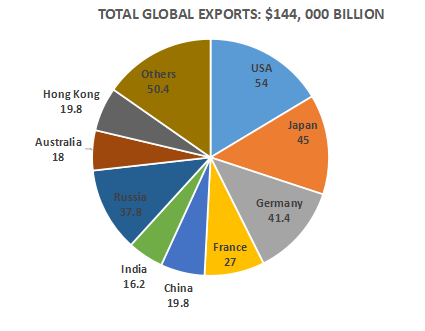# CMAT 2021 Slot 2

Instructions

For the following questions answer them individually

Question 11

Question 12

Question 13

Question 14

Question 15

Question 16

Question 17

Question 18

# A roller is 4m long and has a diameter of 0.7m. It takes exactly 2000 rotations of the roller to level a road. If the cost of using the roller is Rs.4 per square metres, then the total cost of levelling the roadis :Assume, $$\pi=\frac{22}{7}$$

Instructions

Study the pie chart and answer the questions that follow:Note : The value of exports represents the angle corresponding to each country in the pie chart.

Question 19

Question 20

OR